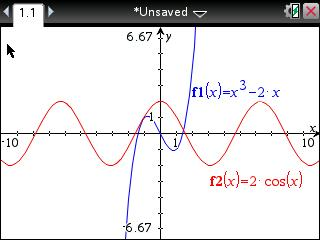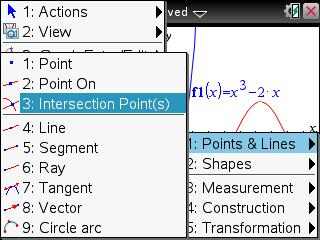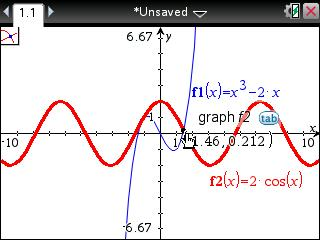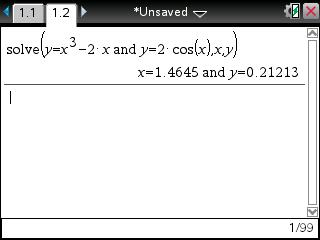# Knowledge Base

## Solution 24790: Simultaneously Solving a System of Nonlinear Equations on the TI-Nspire™ Family Handheld.

### How do I simultaneously solve a system of nonlinear equations on a TI-Nspire family handheld?

Solve a system of nonlinear simultaneous equations on the TI-Nspire family handheld by locating the intersection points on their graphs. The purpose is to solve the system of two equations and two unknowns.  The example below will guide you through solving for the intersection point(s) for x^3-2x=2cos(x) which is the same as the following equations:

y=x^3-2x
y=2cos(x)

Create the graph:

• Use the home key to insert a Graphs document.
• The cursor will be in the entry line for f1(x)=, so press [x] [^]  [-]  [x] [enter] and the first equation will be graphed.
• Press [tab] and the cursor will again be in the entry line at f2(x)=, so press  [cos] [x] and [enter] to produce the second graph
• (Optional and illustrated below) Press [ctrl] [G] to hide the entry line (pressing [ctrl] [G] again will show the entry line.).Define the intersection points:

• Press the menu key and select  Geometry,   Points & Lines, and  Intersection Points.
• Use the navpad keys to move the cursor to the first graph until that graph is highlighted, then press [enter].
• Use the navpad keys to move the cursor to the second graph until it displays as a thick, blinking line and press [enter].
• All intersecting points will be marked with a point (only one point in this case) and its coordinates.This intersecting point represents the simultaneous solution to the two nonlinear equations, x=1.46 and y=0.212.

TI-Nspire CAS

In addition to using graphs, the TI-Nspire CAS handheld can also use the solve( and "and" commands to solve simultaneous equations:

• In the calculator application, press the catalog key.
• Use the tab key to move the cursor to the section titles.
• Use the navpad left and right arrow keys to highlight section 1 and press [enter].
• Press [s] and scroll down to highlight solve(.
• Press [enter] to paste the command into the entry line. Use the and command to complete the simultaneous solution:
• Enter the first equation y=x^3-2x.
• Press the catalog key, then press [a], highlight and, and press [enter] to paste it into the entry line.
• Enter the second equation, y=2cos(x).
• Press [,] [x] [,] [y] and [enter].The solution is x=1.4645 and y=0.21213, the same solution found using graphs.

Texas Instruments recommends all TI-Nspire family users update the handheld and computer software to the latest operating system. More information on the latest operating system can be found at the TI-Nspire and TI-Nspire CAS latest software pages.